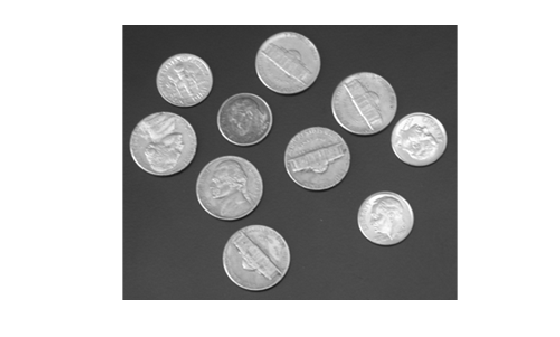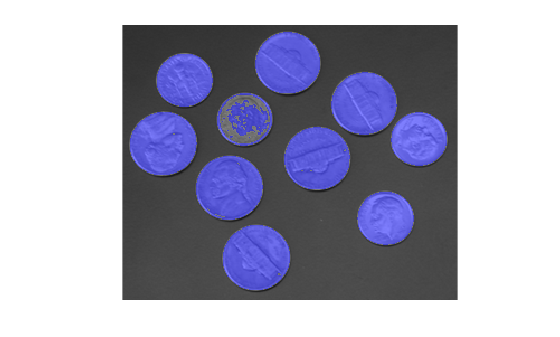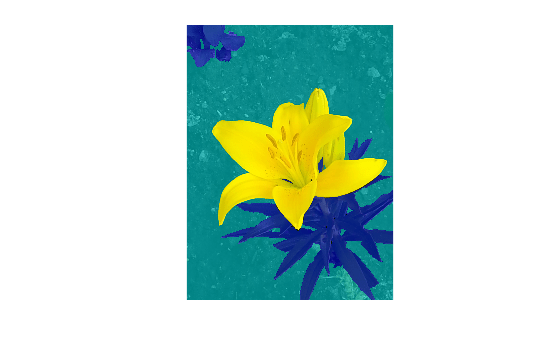# labeloverlay

Overlay label matrix regions on 2-D image

## Syntax

``B = labeloverlay(A,L)``
``B = labeloverlay(A,BW)``
``B = labeloverlay(A,C)``
``B = labeloverlay(___,Name,Value)``

## Description

example

````B = labeloverlay(A,L)` fuses the input image, `A`, with a different color for each nonzero label in label matrix `L`. The `labeloverlay` function does not fuse background pixels with a color.```

example

````B = labeloverlay(A,BW)` fuses the input image with a color where mask `BW` is `true`. The `labeloverlay` function does not fuse background pixels (labeled `false` ) with a color.```
````B = labeloverlay(A,C)` fuses the input image with a different color for each label in categorical matrix `C`. The `labeloverlay` function does not fuse pixels of the `<undefined>` category with a color.```

example

````B = labeloverlay(___,Name,Value)` computes the fused overlay image, `B`, using `Name,Value` pairs to control aspects of the computation.```

## Examples

collapse all

Read an image, then segment it using the `superpixels` function.

```A = imread('kobi.png'); [L,N] = superpixels(A,20);```

Fuse the segmentation results with the original image. Display the fused image.

```B = labeloverlay(A,L); imshow(B)```Read a grayscale image and display it.

```A = imread('coins.png'); imshow(A)```Create a mask using binary thresholding.

```t = graythresh(A); BW = imbinarize(A,t); imshow(BW)```Fuse the mask with the original image. Display the fused image.

```B = labeloverlay(A,BW); imshow(B)```Read a grayscale image.

`A = imread('coins.png');`

Create a mask using binary thresholding.

`BW = imbinarize(A);`

Create categorical labels based on the image contents.

```stringArray = repmat("table",size(BW)); stringArray(BW) = "coin"; categoricalSegmentation = categorical(stringArray);```

Fuse the categorical labels with the original image. Display the fused image.

```B = labeloverlay(A,categoricalSegmentation); imshow(B)```Fuse the original image with only one label from the categorical segmentation. Change the colormap, increase the opacity of the label, and display the result.

```figure C = labeloverlay(A,categoricalSegmentation,'IncludedLabels',"coin", ... 'Colormap','autumn','Transparency',0.25); imshow(C)```## Input Arguments

collapse all

Input image, specified as a 2-D grayscale or color image.

Data Types: `single` | `double` | `int8` | `int16` | `uint8` | `uint16`

Labels, specified as a matrix of nonnegative integers.

Data Types: `single` | `double` | `int8` | `int16` | `int32` | `uint8` | `uint16` | `uint32`

Mask, specified as a logical matrix.

Data Types: `logical`

Category labels, specified as a `categorical` matrix.

Data Types: `categorical`

### Name-Value Arguments

Specify optional pairs of arguments as `Name1=Value1,...,NameN=ValueN`, where `Name` is the argument name and `Value` is the corresponding value. Name-value arguments must appear after other arguments, but the order of the pairs does not matter.

Before R2021a, use commas to separate each name and value, and enclose `Name` in quotes.

Example: `'Colormap','hot'` displays labels in colors from the `'hot'` colormap.

Colormap, specified as the comma-separated pair consisting of `'Colormap'` and one of these values:

• An l-by-3 colormap. RGB triplets in each row of the colormap must be normalized to the range [0, 1]. l is the number of labels in label matrix `L`, binary mask `BW`, or categorical matrix `C`.

• A string or character vector corresponding to one of the valid inputs to the `colormap` function. `labeloverlay` permutes the specified colormap so that adjacent labels are more distinct.

Example: ```[0.2, 0.1, 0.5; 0.1, 0.5, 0.8]```

Example: `'hot'`

Data Types: `single` | `double` | `char` | `string`

Labels to display in the fused image, specified as the comma-separated pair consisting of `'IncludedLabels'` and one of the following:

• An integer, or vector of integers, in the range `[0, max(L(:))]`. By default, `labeloverlay` displays all nonzero labels.

• A string, or vector of strings, corresponding to labels in categorical matrix `C`. By default, `labeloverlay` displays all defined categorical labels.

Any label not included in the vector is considered the background. For example, in the vector `[1,3,4]`, the value 2 would be considered the background, if it existed as a label.

Example: `[1,3,4]`

Example: `["flower","stem"]`

Data Types: `single` | `double` | `int8` | `int16` | `int32` | `uint8` | `uint16` | `uint32` | `string`

Transparency of displayed labels, specified as the comma-separated pair consisting of `'Transparency'` and a number in the range [0, 1].

• A value of `0` makes the colored labels completely opaque.

• A value of `1` makes the colored labels completely transparent.

Data Types: `single` | `double`

## Output Arguments

collapse all

Fused image, returned as a numeric matrix of the same size as `A`.

Data Types: `uint8`

## Version History

Introduced in R2017b